Ch 4. The Mean Value Theorem Multimedia Engineering Math Maximum & Minimum Rolle'sTheorem Mean ValueTheorem MonotonicFunctions First DerivativeTest Concavity &Inflection SecondDerivative Test
 Chapter 1. Limits 2. Derivatives I 3. Derivatives II 4. Mean Value 5. Curve Sketching 6. Integrals 7. Inverse Functions 8. Integration Tech. 9. Integrate App. 10. Parametric Eqs. 11. Polar Coord. 12. Series Appendix Basic Math Units Search eBooks Dynamics Fluids Math Mechanics Statics Thermodynamics Author(s): Hengzhong Wen Chean Chin Ngo Meirong Huang Kurt Gramoll ©Kurt GramollMATHEMATICS - THEORY In this section, Rolle's theorem is introduced along with three applications. Rolle's Theorem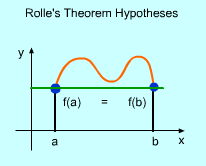Rolle's theorem hypotheses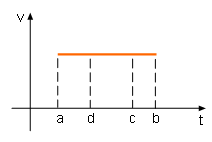Acceleration Equals 0 Rolles's theorem is used to find a function's horizontal tangent line. It is a special case of the mean value theorem which is discussed in the next section. Rolle's theorem states: If a function satisfies the following conditions the function is continuous in a closed interval [a, b], the function is differentiable on this open interval (a, b), the value of the function at the two ends are equal, then there is a point between a and b such that its derivative is 0. This theorem can be better understood by examining the following cases: First, examine the case when the function f(x) is a constant. In this case, df(x)/dx = 0 at all the points from a to b. For example, when a car is driving at an uniform velocity from time a to b, the derivative of the velocity, acceleration, is 0 in this period.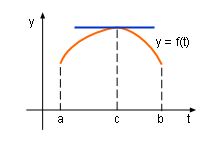Velocity is 0 at the Point c Next, take the case when the function value is larger than the starting point value, f(x) > f(a). In this case the function value is maximum, when df(x)/dx equals 0. For example, when a ball is thrown directly upward, velocity is 0 when the displacement is maximum. In other words, the function maximum will be at point c (see graphic) where the tangent is horizontal.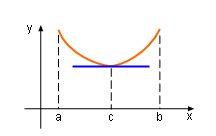df(x)/dx = 0 at Point c Another case is when the function value is smaller than the starting value f(x) < f(a). In this case, df(x)/dx = 0 happens at the minimum value point. For example, the derivative of a hanging cable at its lowest point is 0. At point c (see graphic) the function f(x) is a horizontal tangent line.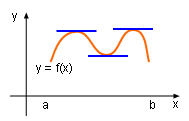Notice that when a function satisfy Rolle's hypotheses, it may have multiple horizontal tangent line at various point when the function local extreme values are reached.